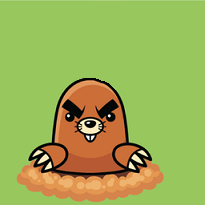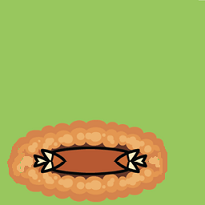# 15.31. A Programming Example¶

Let’s develop a non-trivial GUI program to demonstrate the material presented in the previous lessons. We will develop a GUI Whack-a-mole game where a user tries to click on “moles” as they randomly pop up out of the “ground.”

This discussion will take you through an incremental development cycle that creates a program in well-defined stages. While you might read a finished computer program from “top to bottom,” that is not how it was developed. For a typical GUI program development, you are encouraged to go through these stages:

1. Using scratch paper, physically draw a rough sketch of your user interface.

2. Create the basic structure of your program and create the major frames that will hold the widgets needed for your program’s interface. Give the frames an initial size and color so that you can visually see them, given that there are no widgets inside of them to determine their size.

3. Incrementally add all of the widgets you need for your program and size and position them appropriately.

4. Create your callback functions, stub them out, and assign them to appropriate events. Verify that the events are executing the correct functions.

5. Incrementally implement the functionality needed for each callback function.

When you develop code using incremental development your program should always be executable. You continually add a few lines of code and then test them. If errors occur you almost always know were the errors came from! They came from the lines of code you just added.

## 15.31.1. A Whack-a-mole Game¶

Step 1: Make sure you have a reasonable GUI design and implementation plan before you start coding. Draw a sketch of your initial design on paper and consider how a user will interact with your program.

Step 2: Create the basic structure of your interface using appropriate frame widgets. You will need to give a size to the frames because they will contain no widgets, which is how a frame typically gets its size. It is also suggested that you give each frame a unique color so it is easy to see the area of the window it covers. Here is a basic start for our whack-a-mole game (whack_a_mole_v1.py):

def main():
# Create the entire GUI program
program = WhackAMole()

# Start the GUI event loop
program.window.mainloop()

class WhackAMole:

def __init__(self):
self.window = tk.Tk()
self.mole_frame, self.status_frame = self.create_frames()

def create_frames(self):
mole_frame = tk.Frame(self.window, bg='red', width=300, height=300)
mole_frame.grid(row=1, column=1)

status_frame = tk.Frame(self.window, bg='green', width=100, height=300)
status_frame.grid(row=1, column=2)

return mole_frame, status_frame

if __name__ == "__main__":
main()


Step 3: Incrementally add appropriate widgets to each frame. Don’t attempt to add all the widgets at once. The initial design conceptualized the moles as buttons, so a grid of buttons was added to the left frame, one button for each mole. The exact size of the “mole field” needs to be determined at a future time, so initialize a CONSTANT that can be used to easily change it later. (whack_a_mole_v2.py)import tkinter as tk
from tkinter import PhotoImage

def main():
# Create the entire GUI program
program = WhackAMole()

# Start the GUI event loop
program.window.mainloop()

class WhackAMole:
NUM_MOLES_ACROSS = 4

def __init__(self):
self.window = tk.Tk()
self.mole_frame, self.status_frame = self.create_frames()
self.mole_photo = PhotoImage(file="mole.png")
self.mole_buttons = self.create_moles()

def create_frames(self):
mole_frame = tk.Frame(self.window, bg='red')
mole_frame.grid(row=1, column=1)

status_frame = tk.Frame(self.window, bg='green', width=100)
status_frame.grid(row=1, column=2, sticky=tk.E + tk.W + tk.N + tk.S)

return mole_frame, status_frame

def create_moles(self):
# Source of mole image: https://play.google.com/store/apps/details?id=genergame.molehammer

mole_buttons = []
for r in range(WhackAMole.NUM_MOLES_ACROSS):
row_of_buttons = []
for c in range(WhackAMole.NUM_MOLES_ACROSS):
mole_button = tk.Button(self.mole_frame, image=self.mole_photo)

row_of_buttons.append(mole_button)

mole_buttons.append(row_of_buttons)

return mole_buttons

if __name__ == "__main__":
main()


Continue to add appropriate widgets for the right frame. The final result is shown below, but recognize that it was developed little by little. (whack_a_mole_v3.py)

import tkinter as tk
from tkinter import PhotoImage

def main():
# Create the entire GUI program
program = WhackAMole()

# Start the GUI event loop
program.window.mainloop()

class WhackAMole:
STATUS_BACKGROUND = "white"
NUM_MOLES_ACROSS = 4

def __init__(self):
self.window = tk.Tk()
self.mole_frame, self.status_frame = self.create_frames()
self.mole_photo = PhotoImage(file="mole.png")
self.mole_buttons = self.create_moles()

self.hit_counter, self.miss_counter, self.start_button \
= self.create_status_widgets()

def create_frames(self):
mole_frame = tk.Frame(self.window, bg='red')
mole_frame.grid(row=1, column=1)

status_frame = tk.Frame(self.window, bg=WhackAMole.STATUS_BACKGROUND)
status_frame.grid(row=1, column=2, sticky=tk.N + tk.S + tk.W + tk.W)

return mole_frame, status_frame

def create_moles(self):
# Source of mole image: https://play.google.com/store/apps/details?id=genergame.molehammer

mole_buttons = []
for r in range(WhackAMole.NUM_MOLES_ACROSS):
row_of_buttons = []
for c in range(WhackAMole.NUM_MOLES_ACROSS):
mole_button = tk.Button(self.mole_frame, image=self.mole_photo)

row_of_buttons.append(mole_button)

mole_buttons.append(row_of_buttons)

return mole_buttons

def create_status_widgets(self):
spacer = tk.Label(self.status_frame, text="", bg=WhackAMole.STATUS_BACKGROUND)
spacer.pack(side="top", fill=tk.Y, expand=True)

hit_label = tk.Label(self.status_frame, text="Number of Hits", bg=WhackAMole.STATUS_BACKGROUND)
hit_label.pack(side="top", fill=tk.Y, expand=True)

hit_counter = tk.Label(self.status_frame, text="0", bg=WhackAMole.STATUS_BACKGROUND)
hit_counter.pack(side="top", fill=tk.Y, expand=True)

spacer = tk.Label(self.status_frame, text="", bg=WhackAMole.STATUS_BACKGROUND)
spacer.pack(side="top", fill=tk.Y, expand=True)

miss_label = tk.Label(self.status_frame, text="Number of Misses", bg=WhackAMole.STATUS_BACKGROUND)
miss_label.pack(side="top", fill=tk.Y, expand=True)

miss_counter = tk.Label(self.status_frame, text="0", bg=WhackAMole.STATUS_BACKGROUND)
miss_counter.pack(side="top", fill=tk.Y, expand=True)

spacer = tk.Label(self.status_frame, text="", bg=WhackAMole.STATUS_BACKGROUND)
spacer.pack(side="top", fill=tk.Y, expand=True)

start_button = tk.Button(self.status_frame, text="Start")

spacer = tk.Label(self.status_frame, text="", bg=WhackAMole.STATUS_BACKGROUND)
spacer.pack(side="top", fill=tk.Y, expand=True)

quit_button = tk.Button(self.status_frame, text="Quit")

spacer = tk.Label(self.status_frame, text="", bg=WhackAMole.STATUS_BACKGROUND)
spacer.pack(side="top", fill=tk.Y, expand=True)

return hit_counter, miss_counter, start_button

if __name__ == "__main__":
main()


Step 4: Create a callback function for each event that will cause something to happen in your program. Stub these functions out with a single print statement in each one. Bind an event to each callback function. Now test your program and make sure each event causes the correct print-line in the Python console. (whack_a_mole_v4.py)

import tkinter as tk
from tkinter import PhotoImage

def main():
# Create the entire GUI program
program = WhackAMole()

# Start the GUI event loop
program.window.mainloop()

class WhackAMole():
STATUS_BACKGROUND = "white"
NUM_MOLES_ACROSS = 4

def __init__(self):
self.window = tk.Tk()
self.mole_frame, self.status_frame = self.create_frames()
self.mole_photo = PhotoImage(file="mole.png")
self.mole_buttons = self.create_moles()

self.hit_counter, self.miss_counter, self.start_button, self.quit_button \
= self.create_status_widgets()

self.set_callbacks()

def create_frames(self):
mole_frame = tk.Frame(self.window, bg='red')
mole_frame.grid(row=1, column=1)

status_frame = tk.Frame(self.window, bg=WhackAMole.STATUS_BACKGROUND)
status_frame.grid(row=1, column=2, sticky=tk.E + tk.W + tk.N + tk.S)

return mole_frame, status_frame

def create_moles(self):
# Source of mole image: https://play.google.com/store/apps/details?id=genergame.molehammer

mole_buttons = []
for r in range(WhackAMole.NUM_MOLES_ACROSS):
row_of_buttons = []
for c in range(WhackAMole.NUM_MOLES_ACROSS):
mole_button = tk.Button(self.mole_frame, image=self.mole_photo)

row_of_buttons.append(mole_button)

mole_buttons.append(row_of_buttons)

return mole_buttons

def create_status_widgets(self):
spacer = tk.Label(self.status_frame, text="", bg=WhackAMole.STATUS_BACKGROUND)
spacer.pack(side="top", fill=tk.Y, expand=True)

hit_label = tk.Label(self.status_frame, text="Number of Hits", bg=WhackAMole.STATUS_BACKGROUND)
hit_label.pack(side="top", fill=tk.Y, expand=True)

hit_counter = tk.Label(self.status_frame, text="0", bg=WhackAMole.STATUS_BACKGROUND)
hit_counter.pack(side="top", fill=tk.Y, expand=True)

spacer = tk.Label(self.status_frame, text="", bg=WhackAMole.STATUS_BACKGROUND)
spacer.pack(side="top", fill=tk.Y, expand=True)

miss_label = tk.Label(self.status_frame, text="Number of Misses", bg=WhackAMole.STATUS_BACKGROUND)
miss_label.pack(side="top", fill=tk.Y, expand=True)

miss_counter = tk.Label(self.status_frame, text="0", bg=WhackAMole.STATUS_BACKGROUND)
miss_counter.pack(side="top", fill=tk.Y, expand=True)

spacer = tk.Label(self.status_frame, text="", bg=WhackAMole.STATUS_BACKGROUND)
spacer.pack(side="top", fill=tk.Y, expand=True)

start_button = tk.Button(self.status_frame, text="Start")

spacer = tk.Label(self.status_frame, text="", bg=WhackAMole.STATUS_BACKGROUND)
spacer.pack(side="top", fill=tk.Y, expand=True)

quit_button = tk.Button(self.status_frame, text="Quit")

spacer = tk.Label(self.status_frame, text="", bg=WhackAMole.STATUS_BACKGROUND)
spacer.pack(side="top", fill=tk.Y, expand=True)

return hit_counter, miss_counter, start_button, quit_button

def set_callbacks(self):
# Set the same callback for each mole button
for r in range(WhackAMole.NUM_MOLES_ACROSS):
for c in range(WhackAMole.NUM_MOLES_ACROSS):
self.mole_buttons[r][c]['command'] = self.mole_hit

self.start_button['command'] = self.start
self.quit_button['command'] = self.quit

def mole_hit(self):
print("mole button hit")

def start(self):
print("start button hit")

def quit(self):
print("quit button hit")

if __name__ == "__main__":
main()


Step 5: Add appropriate functionality to the callback functions. This is where the functional logic of your particular application resides. In the case of our whack-a-mole game, we need to be able to count the number of times a user clicks on a mole when it is visible. And we need the moles to appear and disappear at random intervals. Originally each mole was a button widget, but the border around each button was distracting, so they were changed to label widgets. Two images were used to represent a mole: one image is a solid color that matches the frame’s background, and the other image is a picture of a mole. By replacing the image used for each label we can make the moles visible or invisible. A label normally does not have an associated callback, so we bind a left mouse click event, "<ButtonPress-1>" to each label. We can determine whether the mouse click is a “hit” or a “miss” by examining the label under the click to see which image is currently set to the label. We use timer events to change the image on each label. Also notice the use of a messagebox to protect the program from accidental quitting. The end result is shown below. (whack_a_mole_v5.py)

import tkinter as tk
from tkinter import PhotoImage
from tkinter import messagebox
from random import randint

def main():
# Create the entire GUI program
program = WhackAMole()

# Start the GUI event loop
program.window.mainloop()

class WhackAMole:
STATUS_BACKGROUND = "white"
NUM_MOLES_ACROSS = 4
MIN_TIME_DOWN = 1000
MAX_TIME_DOWN = 5000
MIN_TIME_UP = 1000
MAX_TIME_UP = 3000

def __init__(self):
self.window = tk.Tk()
self.window.title("Whack-a-mole")

self.mole_frame, self.status_frame = self.create_frames()

self.mole_photo = PhotoImage(file="mole.png")
self.mole_cover_photo = PhotoImage(file="mole_cover.png")
self.label_timers = {}

self.mole_labels = self.create_moles()

self.hit_counter, self.miss_counter, self.start_button, self.quit_button \
= self.create_status_widgets()

self.set_callbacks()
self.game_is_running = False

def create_frames(self):
mole_frame = tk.Frame(self.window)
mole_frame.grid(row=0, column=0)

status_frame = tk.Frame(self.window, bg=WhackAMole.STATUS_BACKGROUND)
status_frame.grid(row=0, column=1, sticky=tk.E + tk.W + tk.N + tk.S,

return mole_frame, status_frame

def create_moles(self):
# Source of mole image: https://play.google.com/store/apps/details?id=genergame.molehammer

mole_labels = []
for r in range(WhackAMole.NUM_MOLES_ACROSS):
row_of_labels = []
for c in range(WhackAMole.NUM_MOLES_ACROSS):
mole_label = tk.Label(self.mole_frame, image=self.mole_photo)
mole_label.grid(row=r, column=c, sticky=tk.E + tk.W + tk.N + tk.S)
self.label_timers[id(mole_label)] = None

row_of_labels.append(mole_label)

mole_labels.append(row_of_labels)

return mole_labels

def create_status_widgets(self):
spacer = tk.Label(self.status_frame, text="",
bg=WhackAMole.STATUS_BACKGROUND)
spacer.pack(side="top", fill=tk.Y, expand=True)

hit_label = tk.Label(self.status_frame, text="Number of Hits",
bg=WhackAMole.STATUS_BACKGROUND)
hit_label.pack(side="top", fill=tk.Y, expand=True)

hit_counter = tk.Label(self.status_frame, text="0",
bg=WhackAMole.STATUS_BACKGROUND)
hit_counter.pack(side="top", fill=tk.Y, expand=True)

spacer = tk.Label(self.status_frame, text="",
bg=WhackAMole.STATUS_BACKGROUND)
spacer.pack(side="top", fill=tk.Y, expand=True)

miss_label = tk.Label(self.status_frame, text="Number of Misses",
bg=WhackAMole.STATUS_BACKGROUND)
miss_label.pack(side="top", fill=tk.Y, expand=True)

miss_counter = tk.Label(self.status_frame, text="0",
bg=WhackAMole.STATUS_BACKGROUND)
miss_counter.pack(side="top", fill=tk.Y, expand=True)

spacer = tk.Label(self.status_frame, text="",
bg=WhackAMole.STATUS_BACKGROUND)
spacer.pack(side="top", fill=tk.Y, expand=True)

start_button = tk.Button(self.status_frame, text="Start")

spacer = tk.Label(self.status_frame, text="",
bg=WhackAMole.STATUS_BACKGROUND)
spacer.pack(side="top", fill=tk.Y, expand=True)

quit_button = tk.Button(self.status_frame, text="Quit")

spacer = tk.Label(self.status_frame, text="",
bg=WhackAMole.STATUS_BACKGROUND)
spacer.pack(side="top", fill=tk.Y, expand=True)

return hit_counter, miss_counter, start_button, quit_button

def set_callbacks(self):
# Set the same callback for each mole label
for r in range(WhackAMole.NUM_MOLES_ACROSS):
for c in range(WhackAMole.NUM_MOLES_ACROSS):
self.mole_labels[r][c].bind("<ButtonPress-1>", self.mole_hit)

self.start_button['command'] = self.start
self.quit_button['command'] = self.quit

def mole_hit(self, event):

if self.game_is_running:
hit_label = event.widget
if hit_label['image'] == self.mole_cover_photo.name:
# MISSED! Update the miss counter
self.miss_counter['text'] = str(int(self.miss_counter['text']) + 1)
else:
# HIT! Update the hit counter
self.hit_counter['text'] = str(int(self.hit_counter['text']) + 1)
# Remove the mole and don't update the miss counter
self.put_down_mole(hit_label, False)

def start(self):
if self.start_button['text'] == 'Start':
# Change all the mole images to a blank image and
# set a random time for the moles to re-appear on each label.
for r in range(WhackAMole.NUM_MOLES_ACROSS):
for c in range(WhackAMole.NUM_MOLES_ACROSS):
the_label = self.mole_labels[r][c]
the_label['image'] = self.mole_cover_photo
time_down = randint(WhackAMole.MIN_TIME_DOWN,
WhackAMole.MAX_TIME_DOWN)
timer_object = the_label.after(time_down,
self.pop_up_mole, the_label)
self.label_timers[id(the_label)] = timer_object

self.game_is_running = True
self.start_button['text'] = "Stop"

self.hit_counter['text'] = "0"
self.miss_counter['text'] = "0"

else:  # The game is running, so stop the game and reset everything
# Show every mole and stop all the timers
for r in range(WhackAMole.NUM_MOLES_ACROSS):
for c in range(WhackAMole.NUM_MOLES_ACROSS):
the_label = self.mole_labels[r][c]
# Show the mole
the_label['image'] = self.mole_photo
# Delete any timer that is associated with the mole
the_label.after_cancel(self.label_timers[id(the_label)])

self.game_is_running = False
self.start_button['text'] = "Start"

def put_down_mole(self, the_label, timer_expired):

if self.game_is_running:
if timer_expired:
# The mole is going down before it was clicked on, so update the miss counter
self.miss_counter['text'] = str(int(self.miss_counter['text']) + 1)
else:
# The timer did not expire, so manually stop the timer
the_label.after_cancel(self.label_timers[id(the_label)])

# Make the mole invisible
the_label['image'] = self.mole_cover_photo

# Set a call to pop up the mole in the future
time_down = randint(WhackAMole.MIN_TIME_DOWN,
WhackAMole.MAX_TIME_DOWN)
timer_object = the_label.after(time_down, self.pop_up_mole, the_label)
# Remember the timer object so it can be canceled later, if need be
self.label_timers[id(the_label)] = timer_object

def pop_up_mole(self, the_label):
# Show the mole on the screen
the_label['image'] = self.mole_photo

if self.game_is_running:
# Set a call to make the mole disappear in the future
time_up = randint(WhackAMole.MIN_TIME_UP, WhackAMole.MAX_TIME_UP)
timer_object = the_label.after(time_up, self.put_down_mole,
the_label, True)
self.label_timers[id(the_label)] = timer_object

def quit(self):
really_quit = messagebox.askyesno("Quiting?", "Do you really want to quit?")
if really_quit:
self.window.destroy()

if __name__ == "__main__":
main()


## 15.31.2. Summary¶

We developed a complete GUI application in 5 well-designed stages. Hopefully you see the value in incremental software development.

However, the end result is not necessarily easy to understand or modify for future enhancements. The next lesson will introduce a scheme for breaking complete software into more managable pieces.